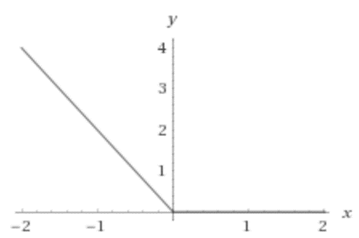# The domain and range of the function and sketch the graph.### Single Variable Calculus: Concepts...

4th Edition
James Stewart
Publisher: Cengage Learning
ISBN: 9781337687805### Single Variable Calculus: Concepts...

4th Edition
James Stewart
Publisher: Cengage Learning
ISBN: 9781337687805

#### Solutions

Chapter 1.1, Problem 42E
To determine

## To find: The domain and range of the function and sketch the graph.

Expert Solution

### Explanation of Solution

Given information:

The given function is,

g(x)=|x|x

Consider the graph of the given function,Since, the function is defined at all real numbers. So, the domain of the given function is all real numbers.

Since, the graph is passing above x axis. So, the range of the function is all non-negative real numbers.

### Have a homework question?

Subscribe to bartleby learn! Ask subject matter experts 30 homework questions each month. Plus, you’ll have access to millions of step-by-step textbook answers!